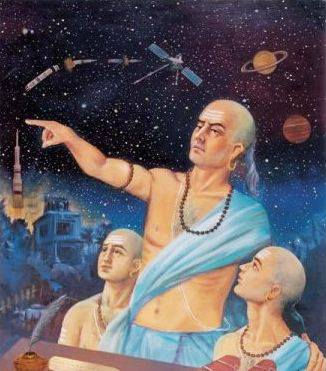top of page# If Aryabhatta invented 0 then how do we know that Ravana had 10 heads?Ravana has 10 head, Kauravas were 100 and Sahastrarjun had 1000 arms years before invention of zero. There were number system in existence much before in all civilisations including India. But there was not zero and its applications.

Brahmi lipi was used widely to write Sanskrit slokas in ancient India. If you see number system in the Brahmi lipi. Here it is…Brahma lipi

Now, if you want to write 11 in brahmi number you have to write the symbol of ten in ten digit and the symbol of one in unit digit.

Here is the number system and it’s representation in Sarada Lipi. (Zero was added in this because it Sarada Lipi was developed in 3rd century.) Similar for Devanagari script which is popularly used to write Hindi and Sanskrit.Aryabhatta invented zero that means he thought that some number like zero exists and one can represent Ten as Symbol of one as ten digit and Symbol of z

ero as unit digit. This was firstly added in Bakhshali Manuscript and then it was added in other Lipis.

Brahmagupta also deserves some credit for invention of zero. Later on Sridharacharya invented the operations of zero in India in 8th century.He clearly mentioned the properties of Zero.

“If zero is added to any number, the sum is the same number; if zero is subtracted from any number, the number remains unchanged; if zero is multiplied by any number, the product is zero”.

So, the conclusion is the zero was invented later than number system. The number system was existed much before and zero was not part of number system then.

## Recent Posts

See All###### on